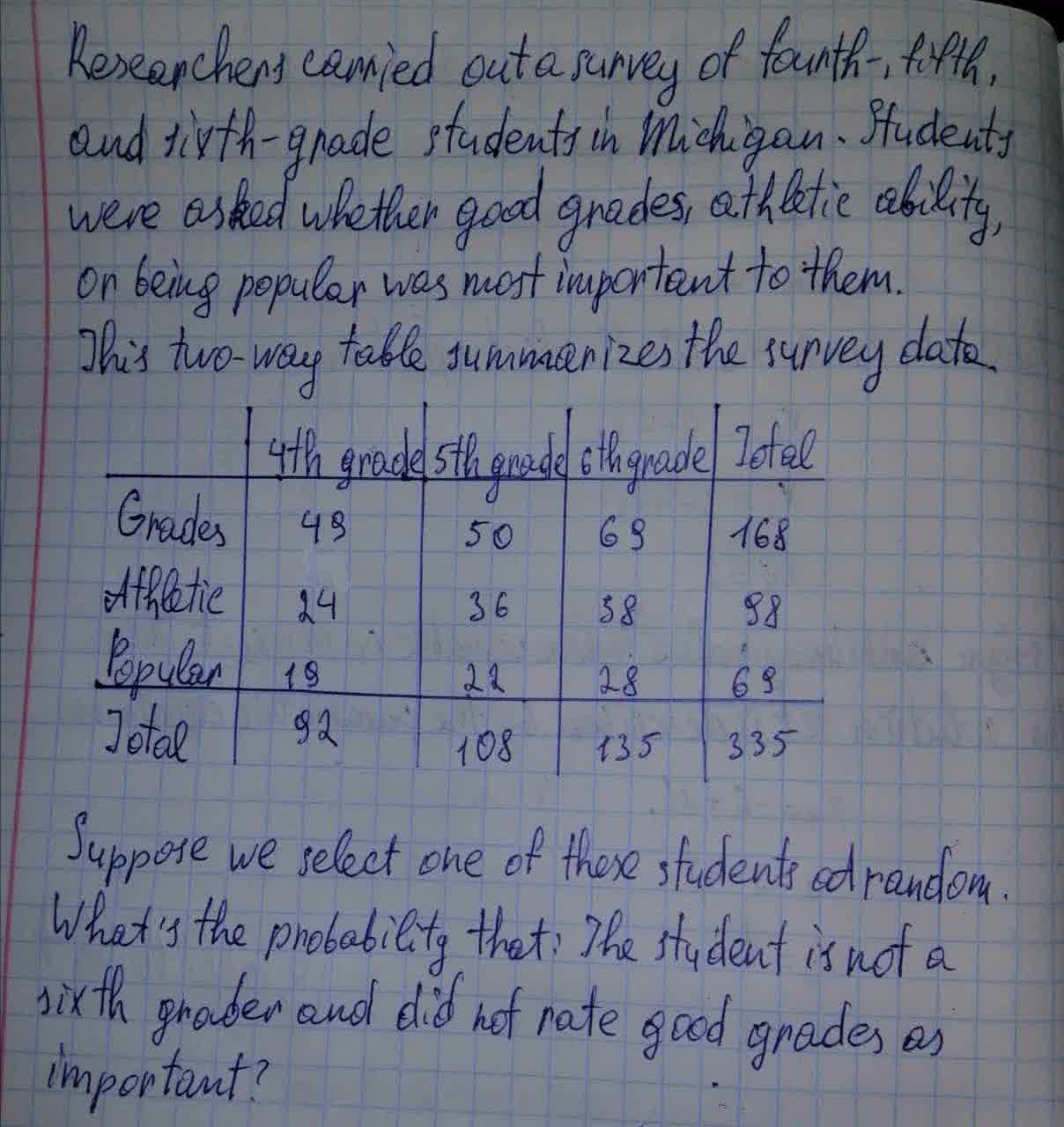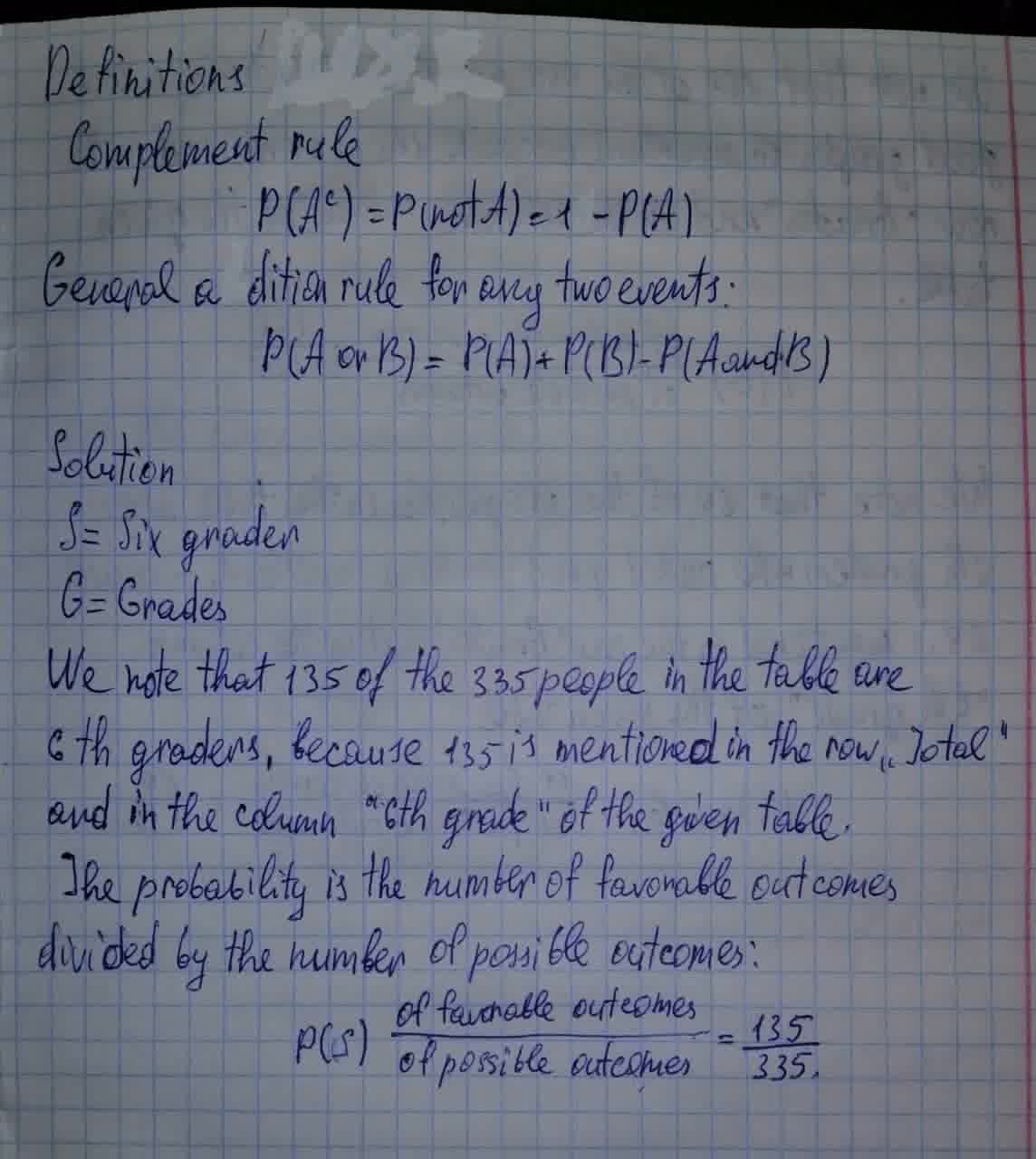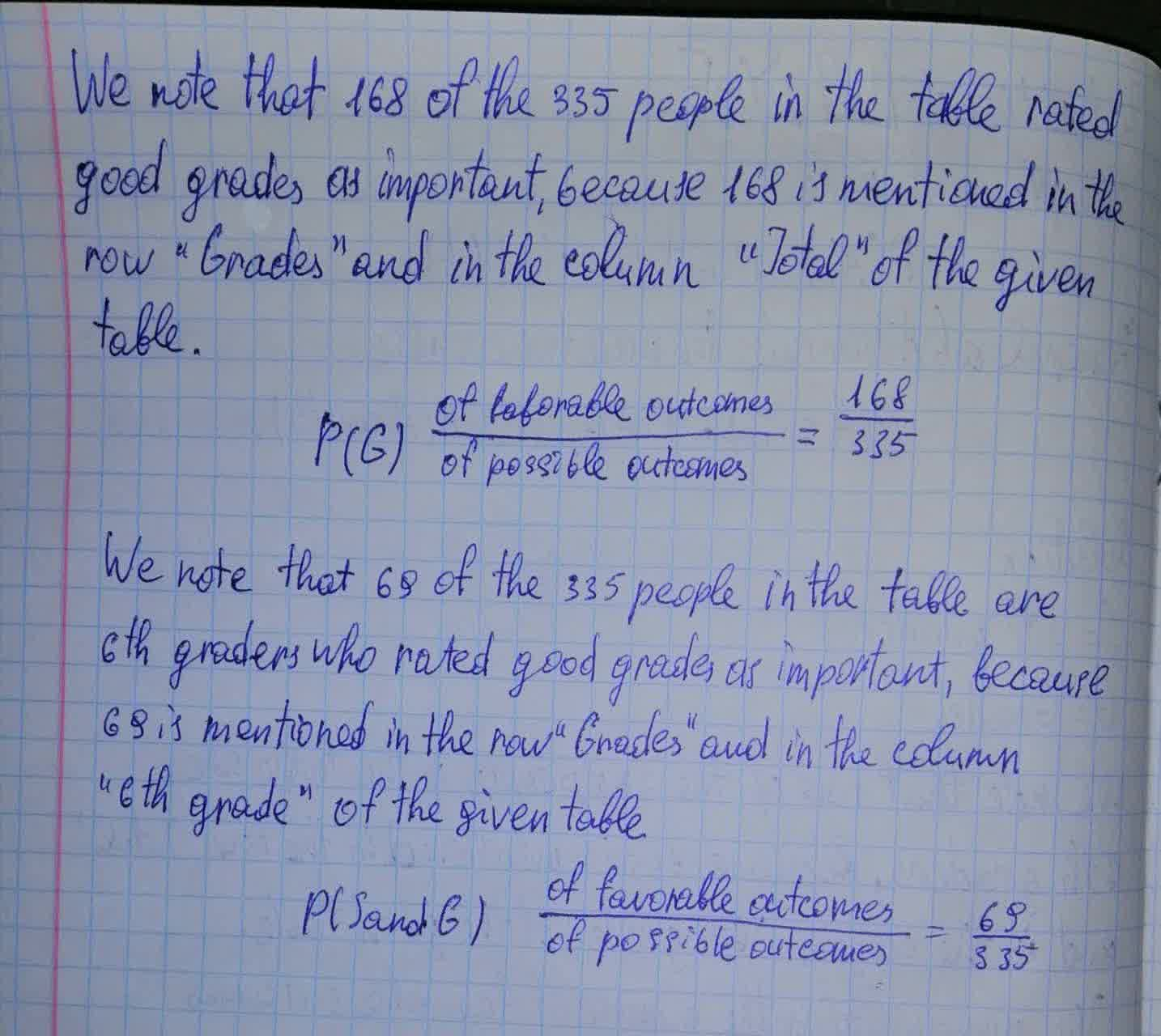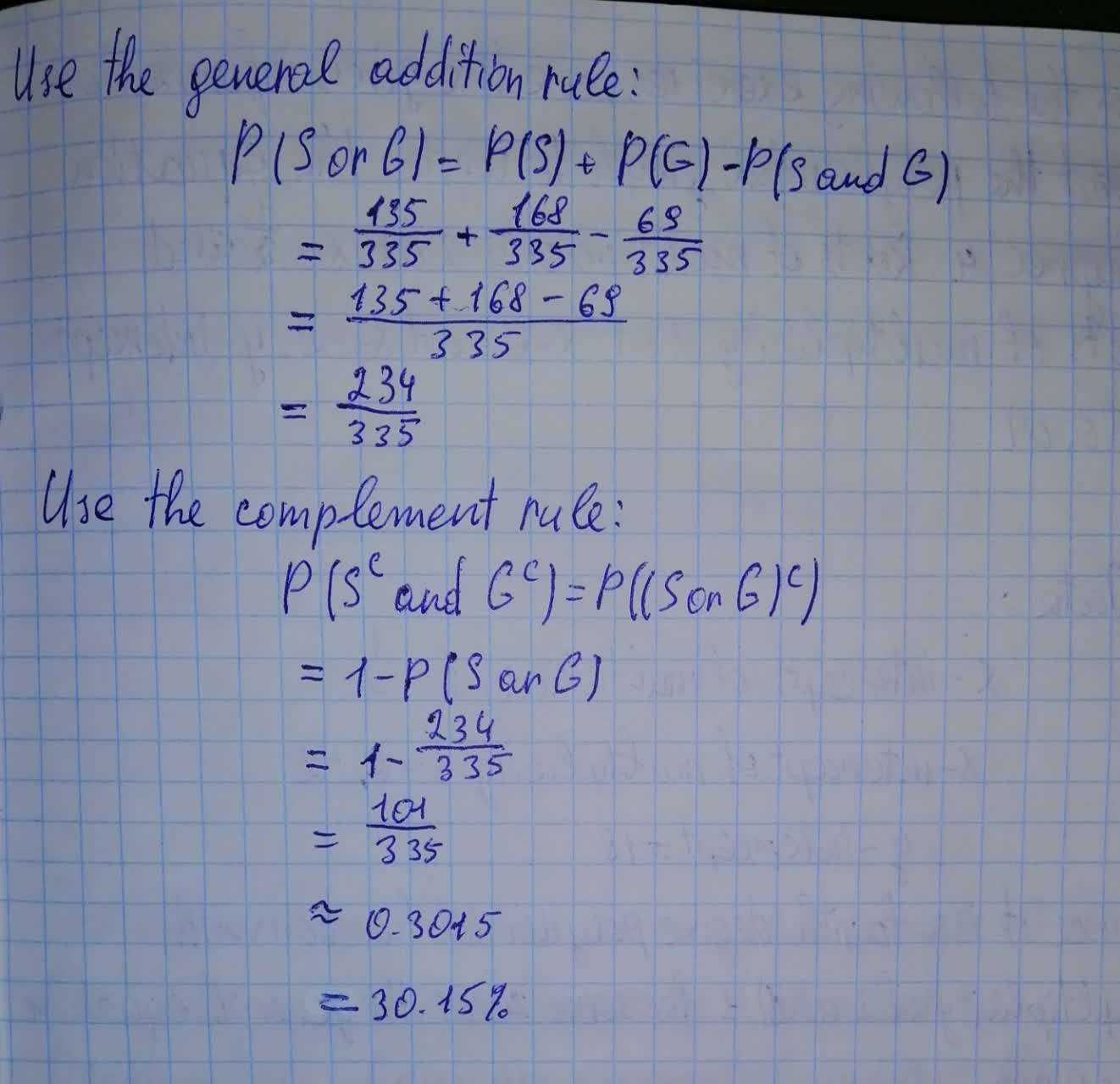# Suppose we select one of these students at random.What's the probability:The student is not a sixth grader and did not rate good grades as important?Cabiolab 2021-08-13 Answered

Researchers carried out a survey of fourth-, fifth-, and sixth-grade students in Michigan. Students were asked whether good grades, athletic ability, or being popular was most important to them. This two-way table summarizes the survey data.
$$\begin{array}{|c|c|}\hline & \text{4th grade} & \text{5th grade} & \text{6th grade} & \text{Total} \\ \hline \text{Grades} & 49 & 50 & 69 & 168 \\ \text{Athletic} & 24 & 36 & 38 & 98 \\ \hline \text{Popular} & 19 & 22 & 28 & 69 \\ \hline \text{Total} & 92 & 108 & 135 & 335 \\ \hline \end{array}$$
Suppose we select one of these students at random. What's the probability that: The student is not a sixth grader and did not rate good grades as important?• Questions are typically answered in as fast as 30 minutes

### Plainmath recommends

• Get a detailed answer even on the hardest topics.
• Ask an expert for a step-by-step guidance to learn to do it yourself.Cristiano Sears

Explanation in the photo below